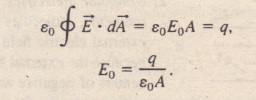# Dielectrics and Gauss Law

Dielectrics and Gauss’ Law

In our discussion of Gauss’ law in Chapter 24, we assumed that the charges existed in a vacuum. Here we shall see how to modify and generalize that law if dielectric materials, such as those listed in Table 26-1, are present.  shows a parallel-plate capacitor of plate area A, both with and without a dielectric. We assume that the charge q on the plates is the same in both situations. Note that the field between the plates induces charges on the faces of the dielectric by one of the methods of Section 76-7. For the situation of  without a dielectric, we can find the electric field Eo between the plates as we  id in  We enclose the charge +q on the top plate with a Gaussian surface and then apply Gauss’ law. Letting Eo represent the magnitude of the field, we find.In with the dielectric in place, we can find the electric field between the plates (and within the dielectric) by using the same Gaussian surface. However, now the surface encloses two types of charge: It still encloses charge +q on the top plate, but it now also encloses the induced charge -q’ on the top face of the dielectric. The charge on the conducting plate is said to be free charge because it can move if we change the electric potential of the plate; the induced charge on the surface of the dielectric is not free charge because it cannot move from that surface. The net charge enclosed by the Gaussian surface in  is q – q’, so  Gauss’ law now gives.1. The flux integral now involves KE, not just E. (The vector eoKE is sometimes called the electric displacement D, so that Eq. 26-34 can be written in the form <j D . iX = q.)

2. The charge q enclosed by the Gaussian surface is now taken to be the free charge only. The induced surface charge is deliberately ignored on the right side of Eq. 26-34, having been taken fulIy into account by introducing the dielectric constant K on the left side

3. Equation 26-34 differs from Eq. 24-7, our original statement of Gauss’ law, only in that eo in the latter equation has been replaced by Keo. We keep K inside the integral of Eq. 26-34 to alIow for cases in which K is not constant over the entire Gaussian surface.

Sample Problem